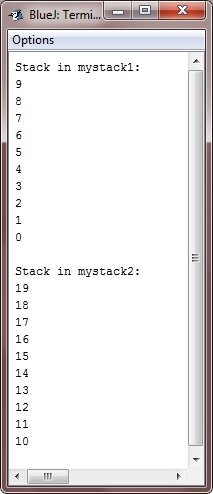# Java Stack

A stack stores data using the first-in, last-out ordering i.e., a stack is like a stack of plates on a table, the first plate put down on the table is the last plate to be used.

## Java Push and Pop

Stacks are controlled through the two operations traditionally called push and pop.

To put an item on the top of the stack, you will use push. And to take an item off the stack, you will use pop.

As you will see, it is easy to encapsulate the entire stack mechanism.

## Java Stack Example

Following is a class called Stack that implements a stack for up to ten integers :

```/* Java Program Example - Java Stack
* This class defines an integer stack that
* can hold 10 integer values
*/

class Stack
{
int stack[] = new int;
int tos;

/* initialize top-of-stack */
Stack()
{
tos = -1;
}

/* push an item onto the stack */
void push(int item)
{
if(tos == 9)
{
System.out.println("Stack is full..!!");
}
else
{
stack[++tos] = item;
}
}

/* pop an item from the stack */
int pop()
{
if(tos < 0)
{
System.out.println("Stack underflow..!!");
return 0;
}
else
{
return stack[tos--];
}
}
}```

As you can see, the Stack class defines two data items and three methods. The stack integers is held by the array stack. This array is indexed by the variable tos, which always contains the index of the top of the stack.

The Stack() constructor initializes tos to -1, which indicates an empty stack. The push() method puts an item on the stack. To retrieve an item, call the pop() method. Since access to the stack is through the push() and the pop(), the fact that the stack is held in an array is actually not relevant to using the stack. For example, the stack could be held in a more complicated data structure, such as a linked list, the interface defined by the push() and pop() would remain the same.

The class TestStack, show below, demonstrates the Stack class. It creates two integer stacks, pushes some values onto each, and then pops them off.

```/* Java Program Example - Java Stack
* The complete version Stack Program */

class Stack
{
int stack[] = new int;
int tos;

/* initialize top-of-stack */
Stack()
{
tos = -1;
}

/* push an item onto the stack */
void push(int item)
{
if(tos == 9)
{
System.out.println("Stack is full..!!");
}
else
{
stack[++tos] = item;
}
}

/* pop an item from the stack */
int pop()
{
if(tos < 0)
{
System.out.println("Stack underflow..!!");
return 0;
}
else
{
return stack[tos--];
}
}
}

class TestStack
{
public static void main(String args[])
{

Stack mystack1 = new Stack();
Stack mystack2 = new Stack();

/* push some numbers onto the stack */
for(int i=0; i<10; i++)
{
mystack1.push(i);
}
for(int j=10; j<20; j++)
{
mystack2.push(j);
}

/* pop those numbers off the stack */
System.out.println("Stack in mystack1 :");
for(int i=0; i<10; i++)
{
System.out.println(mystack1.pop());
}

System.out.println("Stack in mystack2 :");
for(int j=10; j<20; j++)
{
System.out.println(mystack2.pop());
}

}
}```

When the above Java program is compile and executed, it will produce the following output:As you can see, the contents of each stack are separate.

One last point about the Stack class. As it is currently implemented, it is possible for the array that holds the stack, stck, to be altered by the code outside of the Stack class. This leaves the Stack open to misuse or mischief.

Tools
Calculator